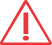You are here: Home > Practicals > Double Ball Bounce
Practicals
Categories
Physics
- Forces
Options

# Double Ball Bounce AKA: Conservation of Momentum

## Summary

Two balls are dropped, one on top of the other. As they hit the floor, the ball on top bounces much higher than it will on its own.

## Operation

This is a demonstration of a physics principle called the 'conservation of momentum'. It involves taking two balls, one large (a basketball or football) and one small (a tennis ball) and holding them so that the tennis ball is directly on top of the basketball. They are dropped at the same time from a height of a metre or so. As the basketball hits the ground, it bounces. The smaller ball collides with the upward travelling basketball, making it bounce much higher than it would have done on its own.

Each ball is usually bounced separately first and the height of the bounce measured. Because energy is lost through sound, heat etc as a ball hits the floor it will never bounce to, or higher than, the height it is dropped from. That is what makes this demonstration interesting as the tennis ball will travel higher than from where it was dropped from when combined with the bounce of the basketball.

Equipment required (per demonstration):

• tennis ball
• metre rule (optional)
The reason the tennis ball bounces high is because of the difference in masses of the two objects. The Gravitational Potential Energy (GPE) of each ball is related to its weight and the height it is lifted to, using the formula:

GPE = mgh

Where m=mass, g=acceleration due to gravity and h=height.

The conservation of momentum law can be stated as:

For a collision occurring between object 'A' and object 'B', the total momentum of the two objects before the collision is equal to the total momentum of the two objects after the collision. The momentum lost by object A is equal to the momentum gained by object B.

Applying this to the demonstration, When the two collide, their momentums are the same but because the larger ball has a greater mass, to balance the equation the smaller ball must achieve a greater velocity. The result of this is that the tennis ball bounces very high.

Typical technician preparation time for this demonstration: 5 minutes.

## SafetyThe contents of this page are for information only. Please refer to CLEAPSS, SSERC or ASE safety advice and/or publications before undertaking any preparation, practical experiment or using any equipment featured on this site or any other.